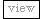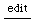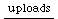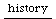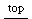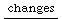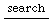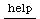## evaluation dynamics of functions

The problem discussed here is a special view on Discrete vs. Continuous Evaluation, it is a common source for misconceptions. I now found out that it is properly defined as destructive / nondestructive assignment.
Some clarification on this subject were found when discussing assignment methods : Understanding Assignment and side effect

the start is a simple modification of an object:
```var object, function, result
result = function.value(object)
```

now the question is when you change object, in what way will result change?
• if it doesn't change I'd call function referential opaque regarding this object
• if it changes it can be called referentially transparent

Connected to this is the question when is some code evaluated?
• at compile time on startup
• by the interpreter when starting the scripted code
• at a certain point in time
• at control rate
• at audio rate

A typical problem would be the reason why to use a Plug UGen.
A Plug makes many functions referentially transparent and by using the source method

```compile this:
(
{
a = 100;
b = SinOsc.ar(a, 0, 0.1)
}.play;
)

and while it is running, compile something like this:
a = 2000;

compare to this:
(
{
a = Plug.kr(100);

b = SinOsc.ar(a, 0, 0.1)
}.play;
)

a.source = 2000;

```

in the second case I would say that SinOsc.ar(Plug.kr(a)) is referentially transparent regarding a,
whereas SinOsc.ar(a) is not referentially transparent regarding a (a be an integer or a float)

of course the outcome will vary depending on the context. One example is:
```(
{
Spawn.ar({ 	a = SinOsc.ar(rrand(100, 200.0));
a &star; Line.kr(0.1, 0, 1) }, 2, 2)
}.play
)
```

and:

(
{
a = SinOsc.ar(rrand(100, 200.0));
Spawn.ar({ a * Line.kr(0.1, 0, 1) }, 2, 2)
}.play
)

authors so far:
jrh